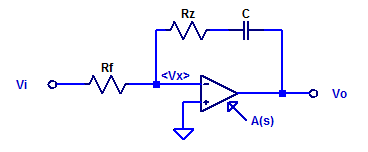# Low-Noise, High-PSRR LDO – Error Amplifier

In this post we will develop and analyze a practical “type 2” error amplifier. The distinction of “type” 1, 2, or 3, is merely a description of how many poles are present in the compensator. All three types of error amplifiers will attempt to employ a pole at DC, i.e. operate as an integrator at low frequencies. Of course an error amplifier with an ideal integrator has a steady-state error of zero. However as is almost to all to common, digi-key does not stock any textbook class op-amps, with infinite differential gain and endless amounts of bandwidth.

The figure below illustrates a practical type 2 error amplifier with voltage input and voltage output.The output is sensed via feedback resistor $$R_f$$ and the set-point is provided by voltage source $$V_{REF}$$ to the positive input terminal of amplifier U1.

## Transfer Function

The voltage reference sets the DC operating point but its value has no impact on loop dynamics (provided its within the common-mode of the error amplifier). As a result, the schematic can be simplified as shown in  the figure below to develop the transfer function.We first note that the the amplifier is non-ideal, having its own open-loop transfer function $$A(s)$$. Provided one employs an op-amp which is advertised as being unity-gain stable (most are), a simplified model for $$A(s)$$ is the following,

$A(s) = \dfrac{A_{ol}}{1-\dfrac{s}{p_a}}$

where the single dominant pole occurs at

\begin{align*}
p_a &= -\dfrac{\text{GBWP}}{A_{ol}} \;\; [\text{Hz}] \\
p_a &= -\dfrac{\text{GBWP}}{A_{ol}} 2\pi \;\; [\text{rad/s}]
\end{align*}

We can relate the input terminal potential of the opamp to its output as
$V_o = – V_x A(s)$
Note the negative as the input is on the inverting terminal.

Applying KCL at the intermediate node $$V_x$$ as,

$\dfrac{V_x-V_i}{R_f} + \dfrac{V_x-V_o}{R_z + 1/sC} = 0$

$V_x\left( \dfrac{1}{R_f} + \dfrac{1}{R_z+1/sC} \right) – \dfrac{V_i}{R_f} – \dfrac{V_o}{R_z + 1/sC} = 0$

Substituting the op-amps open-loop gain yeilds,

\begin{align*}
V_x &= \dfrac{-V_o}{A(s)}\\
V_x &= \dfrac{-V_o\left( 1 – s/p_a\right)}{A_{ol}}
\end{align*}

Substitution of $$V_x$$,

$\dfrac{-V_o\left( 1 – s/p_a\right)}{A_{ol}} \left( \dfrac{R_f+R_z+1/sC}{R_f(R_z+1/sC)} \right) – \dfrac{V_o}{R_z + 1/sC} = \dfrac{V_i}{R_f}$

$\dfrac{-V_o}{R_f(R_z+1/sC)} \left( \dfrac{\left( 1 – s/p_a\right)\left(R_f+R_z+1/sC\right)}{A_{ol}} -R_f\right) = \dfrac{V_i}{R_f}$

$V_o\left( \dfrac{\left( 1 – s/p_a\right)\left(R_f+R_z+1/sC\right) + A_{ol}R_f}{A_{ol}} \right) = -V_i(R_z+1/sC)$

Finally the transfer function becomes,

\begin{align*}
\dfrac{V_o}{V_i} &= \dfrac{-A_{ol}(R_z+1/sC)}{A_{ol}R_f + R_f + R_z – \dfrac{1}{Cp_a} + \dfrac{1}{sC} – \dfrac{s(R_f+R_z)}{p_a} } \\ \\
H(s) &= \dfrac{-A_{ol}(1 + sR_zC)}{1 + s\left( -1/p_a +C\left( R_f + R_z + A_{ol}R_f \right)\right) – \dfrac{s^2C(R_f+R_z)}{p_a} } \\
\end{align*}

The one zero occurs at
$z_1 = \dfrac{-1}{R_zC}$

If we assume the poles are well separated, the dominate pole can be approximated as,
$p_1 \simeq \dfrac{-1}{-1/p_a +C\left( R_f + R_z + A_{ol}R_f \right)}$
The dominate pole can be further simplified as,

$p_1 \simeq \dfrac{-1}{A_{ol}R_fC}$

However $$|p_1|$$ can never be greater than the intrinsic op-amp pole $$|p_a|$$.

The second pole then occurs at,
$p_2 = \dfrac{p_aA_{ol}R_fC}{C(R_z+R_f)}$

$p_2 = A_{ol}\left( \dfrac{R_f}{R_z+R_f} \right) p_a$

The approximate transfer function is then,

$H(s) = \dfrac{-A_{ol}(1 – s/z_1)}{(1 – s/p_1)(1 – s/p_2)}$

## Amplifier Limitations

For the purposes of discussion, consider an error amplifier with the following parameters,

\begin{align*}
R_f &= 2 \text{ k}\Omega \\
R_z &= 100 \text{ k}\Omega \\
C &= 628 \text{ pF} \\
A_{ol} &= 100 \text{ dB} \\
\text{GBWP} &= 10 \text{ MHz} \\
\end{align*}

The exact transfer function is,

$H(s) = \dfrac{-(100000+ 6.28 s )}{1+0.1273 s+1.019\text{e-}007 s^2 }$

With poles and a zero of

$z_1 = -2.534 \text{ [kHz]},\;\;\; p_1 = -1.251 \text{ [Hz]},\;\;\; p_2 = -198.7\text{ [kHz]}$

The approximated poles and zero as developed above is the following

$\hat{z_1} = -2.534 \text{ [kHz]},\;\;\; \hat{p_1} = -1.267 \text{ [Hz]},\;\;\; \hat{p_2} = -196.1\text{ [kHz]}$

A bode plot of the transfer function of this sample type 2 error amplifier is shown in the figure below. Additionally the open-loop gain of the employed op-amp $$A(s)$$ is also included.We can see that, the blue trace $$H(s)$$, resembles the classic type 2 error amplifier as having a pole near DC, and a mid-band region of phase boost. However it should also be apparent that the error amplifier cannot provide a response greater than that of the open-loop gain of the op-amp $$A(s)$$. As a result, we can not actually place a pole at DC, as we would require an op-amp with infinite open-loop gain $$A_{ol}$$.

We are free to choose an arbitrary location of the zero of the error amplifier. Following a choice for $$z_1$$ we may choose only one of the following secondarily,

– Pole location $$p_1$$
– Pole location $$p_2$$
– Error amplifier gain during phase boost

The two hallmark specifications of an op-amp regarding loop dynamics are open-loop gain $$A_{ol}$$ and Gain-BandWidth product. A typical open-loop gain for an op-amp is 100 dB, however some are available with 120 dB or even 150 dB of open-loop gain. The GBWP is also the unity gain crossover frequency of the amplifier, which declines at a rate of 20 dB/dec for the single pole model.

This post focused on loop dynamics, however as will be seen in a future post, a plethora of other op-amp specifications are also important for its steady state and dynamic performance, such as input offset voltage, common-mode rejection, power-supply rejection, temperature coefficients, input-bias currents, input-referred noise, and others…

## 1 thought on “Low-Noise, High-PSRR LDO – Error Amplifier”

1.kézműves Ajándék says:

Kiváló webhely van itt… Manapság nehéz olyan jó minőségű írást találni, mint az Öné.

Nagyon nagyra értékelem az olyan egyéneket, mint te!